login  home  contents  what's new  discussion  bug reports help  links  subscribe  changes  refresh  edit

Ref:

http://en.wikipedia.org/wiki/Cayley%E2%80%93Dickson_construction

"The Cayley–Dickson construction, named after Arthur Cayley and Leonard Eugene Dickson, produces a sequence of algebras over the field of real numbers, each with twice the dimension of the previous one. The algebras produced by this process are known as Cayley–Dickson algebras; since they extend the complex numbers, ... "

complex numbers, quaternions, octonions, sedenions, ...

http://en.wikipedia.org/wiki/Hypercomplex_number

fricas
(1) -> <spad>
fricas
)abbrev domain CALEY CaleyDickson
CaleyDickson(C:CommutativeRing,gen:Symbol,gamma:C):ComplexCategory(C) with
hyper:List % -> %
++ convert a list of scalars to a hyper-comnplex number
scalars: % -> List %
++ convert a hyper-complex number to a list of scalars
Rep := Record(re:C, im:C)
rep(x:%):Rep == x pretend Rep
per(x:Rep):% == x pretend %
complex(x:C,y:C):% == per [x,y]
real(x:%):C == rep(x).re
imag(x:%):C == rep(x).im
--mul(x:C,y:%):% == complex(x * real y, x * imag y)
mul(x:C,y:%):% == per [x * rep(y).re, x * rep(y).im]
-- Many funtctions are inherited from ComplexCategory
--
-- To Do:
-- 1) Check which functions are still correct for higher-order algebras!
0:% == complex(0,0)
--zero?(x:%):Boolean == zero? real x and zero? imag x
zero?(x:%):Boolean == x = 0
1:% == complex(1,0)
--one?(x:%):Boolean  == one? real x and zero? imag x
one?(x:%):Boolean == x = 1
if C has conjugate:C->C then
-- In general we need conjugate
(x:% * y:%):% ==
complex(real x * real y - gamma*conjugate imag y * imag x,
imag y * real x + imag x * conjugate real y)
conjugate(x:%):% == complex(conjugate(real x), -imag x)
else
-- If not complex then conjugate is identity
(x:% * y:%):% ==
complex(real x * real y - gamma*imag y * imag x,
imag y * real x + imag x * real y)
conjugate(x:%):% == complex(real x, -imag x)
-- correct order
norm(x:%):C == retract(conjugate(x)*x)
if C has Field then
inv(x:%):% == mul(inv norm x, conjugate x)
(x:% / y:%):% == x * inv(y)
if C has rank:()->PositiveInteger then
rank():PositiveInteger == 2*rank()$C else rank():PositiveInteger == 2 if C has basis:()->Vector C then basis():Vector % == concat([complex(i,0) for i in entries basis()$C],
[complex(0,i) for i in entries basis()$C]) else basis():Vector % == [1,imaginary()] if C has scalars: C -> List C then scalars(x:%):List % == map(coerce,concat(scalars real x, scalars imag x))$ListFunctions2(C,%)
else
scalars(x:%):List % == [ coerce real x, coerce imag x ]
if C has hyper:List C -> C then
hyper(x:List %):% ==
h:Integer := divide(#x,2).quotient
complex(hyper([retract(x.i)@C for i in 1..h]),hyper([retract(x.i)@C for i in h+1..#x]))
else
hyper(x:List %):% == complex(retract x.1,retract x.2)
coerce(x:%):OutputForm ==
outr:=real(x)::OutputForm
imag x = 0 => return outr
outi := hconcat(imag(x)::OutputForm, gen::OutputForm)
if imag x = 1 then
outi := gen::OutputForm
if imag x = -1 then
outi := -(gen::OutputForm)
if C has imaginary:()->C then
if imag x = -imaginary()$C then outi := -hconcat(imaginary()$C::OutputForm,gen::OutputForm)
real x = 0 => return outi
return outr + outi</spad>
fricas
Compiling FriCAS source code from file
using old system compiler.
CALEY abbreviates domain CaleyDickson
------------------------------------------------------------------------
initializing NRLIB CALEY for CaleyDickson
compiling into NRLIB CALEY
****** Domain: C already in scope
compiling local rep : $-> Rep CALEY;rep is replaced by x Time: 0.05 SEC. compiling local per : Rep ->$
CALEY;per is replaced by x
Time: 0 SEC.
compiling exported complex : (C,C) -> $Time: 0 SEC. compiling exported real :$ -> C
Time: 0 SEC.
compiling exported imag : $-> C Time: 0 SEC. compiling local mul : (C,$) -> $Time: 0 SEC. compiling exported Zero : () ->$
Time: 0 SEC.
compiling exported zero? : $-> Boolean Time: 0 SEC. compiling exported One : () ->$
Time: 0 SEC.
compiling exported one? : $-> Boolean Time: 0 SEC. augmenting C: (SIGNATURE C conjugate (C C)) compiling exported * : ($,$) ->$
Time: 0.01 SEC.
compiling exported conjugate : $->$
Time: 0 SEC.
compiling exported * : ($,$) -> $Time: 0 SEC. compiling exported conjugate :$ -> $Time: 0 SEC. compiling exported norm :$ -> C
Time: 0 SEC.
****** Domain: C already in scope
augmenting C: (Field)
compiling exported inv : $->$
Time: 0 SEC.
compiling exported / : ($,$) -> $Time: 0 SEC. augmenting C: (SIGNATURE C rank ((PositiveInteger))) compiling exported rank : () -> PositiveInteger Time: 0.01 SEC. compiling exported rank : () -> PositiveInteger CALEY;rank;Pi;19 is replaced by 2 Time: 0 SEC. augmenting C: (SIGNATURE C basis ((Vector C))) compiling exported basis : () -> Vector$
Time: 0.01 SEC.
compiling exported basis : () -> Vector $Time: 0 SEC. augmenting C: (SIGNATURE C scalars ((List C) C)) compiling exported scalars :$ -> List $Time: 0.01 SEC. compiling exported scalars :$ -> List $Time: 0 SEC. augmenting C: (SIGNATURE C hyper (C (List C))) compiling exported hyper : List$ -> $Time: 0.02 SEC. compiling exported hyper : List$ -> $Time: 0 SEC. compiling exported coerce :$ -> OutputForm
augmenting C: (SIGNATURE C imaginary (C))
Time: 0.01 SEC.
****** Domain: C already in scope
****** Domain: C already in scope
augmenting C: (TranscendentalFunctionCategory)
****** Domain: C already in scope
augmenting C: (RealNumberSystem)
****** Domain: C already in scope
augmenting C: (TranscendentalFunctionCategory)
****** Domain: C already in scope
augmenting C: (Comparable)
****** Domain: C already in scope
augmenting C: (ConvertibleTo (InputForm))
****** Domain: C already in scope
augmenting C: (ConvertibleTo (Pattern (Float)))
****** Domain: C already in scope
augmenting C: (ConvertibleTo (Pattern (Integer)))
****** Domain: C already in scope
augmenting C: (DifferentialRing)
****** Domain: C already in scope
augmenting C: (Eltable C C)
****** Domain: C already in scope
augmenting C: (Evalable C)
****** Domain: C already in scope
augmenting C: (Field)
****** Domain: C already in scope
augmenting C: (Finite)
****** Domain: C already in scope
augmenting C: (FiniteFieldCategory)
****** Domain: C already in scope
augmenting C: (InnerEvalable (Symbol) C)
****** Domain: C already in scope
augmenting C: (IntegerNumberSystem)
****** Domain: C already in scope
augmenting C: (IntegralDomain)
****** Domain: C already in scope
augmenting C: (LinearlyExplicitOver (Integer))
****** Domain: C already in scope
augmenting C: (PartialDifferentialRing (Symbol))
****** Domain: C already in scope
augmenting C: (PatternMatchable (Float))
****** Domain: C already in scope
augmenting C: (PatternMatchable (Integer))
****** Domain: C already in scope
augmenting C: (RealConstant)
****** Domain: C already in scope
augmenting C: (RealNumberSystem)
****** Domain: C already in scope
augmenting C: (RetractableTo (Fraction (Integer)))
****** Domain: C already in scope
augmenting C: (RetractableTo (Integer))
****** Domain: C already in scope
augmenting C: (TranscendentalFunctionCategory)
(time taken in buildFunctor:  250)
;;;     ***       |CaleyDickson| REDEFINED
;;;     ***       |CaleyDickson| REDEFINED
Time: 0.30 SEC.
Warnings:
 rep: pretendRep -- should replace by @
 per: pretend$-- should replace by @  real: re has no value  imag: im has no value  mul: re has no value  mul: im has no value  scalars:$$has no value Cumulative Statistics for Constructor CaleyDickson Time: 0.42 seconds finalizing NRLIB CALEY Processing CaleyDickson for Browser database: --->-->CaleyDickson(constructor): Not documented!!!! --------(hyper (% (List %)))--------- --->-->CaleyDickson((hyper (% (List %)))): Improper first word in comments: convert "convert a list of scalars to a hyper-comnplex number" --------(scalars ((List %) %))--------- --->-->CaleyDickson((scalars ((List %) %))): Improper first word in comments: convert "convert a hyper-complex number to a list of scalars" --->-->CaleyDickson(): Missing Description ; compiling file "/var/aw/var/LatexWiki/CALEY.NRLIB/CALEY.lsp" (written 09 OCT 2022 07:51:53 PM): ; /var/aw/var/LatexWiki/CALEY.NRLIB/CALEY.fasl written ; compilation finished in 0:00:00.131 ------------------------------------------------------------------------ CaleyDickson is now explicitly exposed in frame initial CaleyDickson will be automatically loaded when needed from /var/aw/var/LatexWiki/CALEY.NRLIB/CALEY Test fricas R := FRAC POLY Integer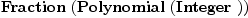(1) Type: Type Complex Numbers fricas C := CaleyDickson(R,'i,1)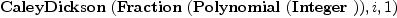(2) Type: Type fricas rank()$C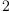(3)
Type: PositiveInteger?
fricas
Ce:ILIST(C,0) := construct entries basis()$C(4) Type: IndexedList?(CaleyDickson(Fraction(Polynomial(Integer)),i,1),0) fricas matrix [[Ce.i * Ce.j for j in 0..#Ce-1] for i in 0..#Ce-1]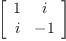(5) Type: Matrix(CaleyDickson(Fraction(Polynomial(Integer)),i,1)) fricas -- -- compare -- Cg:ILIST(Complex R,0) := construct map(x+-> complex(x.1,x.2), 1$SquareMatrix(2,FRAC INT)::List List FRAC INT)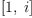(6)
Type: IndexedList?(Complex(Fraction(Polynomial(Integer))),0)
fricas
matrix [[Cg.i * Cg.j for j in 0..#Cg-1] for i in 0..#Cg-1](7)
Type: Matrix(Complex(Fraction(Polynomial(Integer))))
fricas
-- normed?
c1:C := hyper [subscript('c1,[i]) for i in 1..2]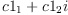(8)
Type: CaleyDickson(Fraction(Polynomial(Integer)),i,1)
fricas
c2:C := hyper [subscript('c2,[i]) for i in 1..2]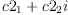(9)
Type: CaleyDickson(Fraction(Polynomial(Integer)),i,1)
fricas
c3:C := hyper [subscript('c3,[i]) for i in 1..8]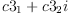(10)
Type: CaleyDickson(Fraction(Polynomial(Integer)),i,1)
fricas
-- Normed?
test(norm(c1*c2)=norm(c1)*norm(c2))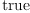(11)
Type: Boolean
fricas
-- Commutative?
test( c1 * c2 = c2 * c1 )(12)
Type: Boolean
fricas
-- Associative?
test((c1 * c2) * c3 = c1 * (c2 * c3))(13)
Type: Boolean
fricas
-- inverse
c1inv := inv c1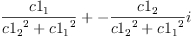(14)
Type: CaleyDickson(Fraction(Polynomial(Integer)),i,1)
fricas
test(c1 * c1inv = 1)(15)
Type: Boolean

Quaternions

fricas
Q := CaleyDickson(C,'j,1)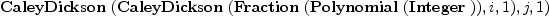(16)
Type: Type
fricas
rank()$Q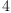(17) Type: PositiveInteger? fricas Qe:ILIST(Q,0) := construct entries basis()$Q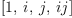(18)
Type: IndexedList?(CaleyDickson(CaleyDickson(Fraction(Polynomial(Integer)),i,1),j,1),0)
fricas
matrix [[Qe.i * Qe.j for j in 0..#Qe-1] for i in 0..#Qe-1]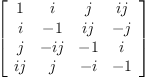(19)
Type: Matrix(CaleyDickson(CaleyDickson(Fraction(Polynomial(Integer)),i,1),j,1))
fricas
--
-- compare
--
Qg:ILIST(Quaternion R,0) := construct map(x+-> quatern(x.1,x.2,x.3,x.4),
1$SquareMatrix(4,FRAC INT)::List List FRAC INT)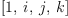(20) Type: IndexedList?(Quaternion(Fraction(Polynomial(Integer))),0) fricas matrix [[Qg.i * Qg.j for j in 0..#Qg-1] for i in 0..#Qg-1]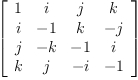(21) Type: Matrix(Quaternion(Fraction(Polynomial(Integer)))) fricas q1:Q := hyper [subscript('q1,[i]) for i in 1..4]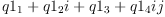(22) Type: CaleyDickson(CaleyDickson(Fraction(Polynomial(Integer)),i,1),j,1) fricas q2:Q := hyper [subscript('q2,[i]) for i in 1..4]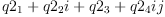(23) Type: CaleyDickson(CaleyDickson(Fraction(Polynomial(Integer)),i,1),j,1) fricas q3:Q := hyper [subscript('q3,[i]) for i in 1..8]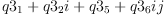(24) Type: CaleyDickson(CaleyDickson(Fraction(Polynomial(Integer)),i,1),j,1) fricas -- Normed? test( norm(q1*q2) = norm(q1)*norm(q2))(25) Type: Boolean fricas -- Commutative? test( q1 * q2 = q2 * q1 )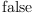(26) Type: Boolean fricas -- Associative? test((q1 * q2) * q3 = q1 * (q2 * q3))(27) Type: Boolean fricas -- inverse q1inv := inv q1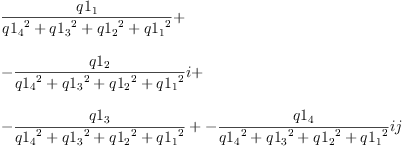(28) Type: CaleyDickson(CaleyDickson(Fraction(Polynomial(Integer)),i,1),j,1) fricas test(q1 * q1inv = 1)(29) Type: Boolean Octonions fricas O:=CaleyDickson(Q,'k,1)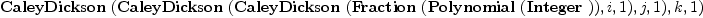(30) Type: Type fricas rank()$O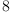(31)
Type: PositiveInteger?
fricas
Oe:ILIST(O,0) := construct entries basis()$O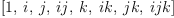(32) Type: IndexedList?(CaleyDickson(CaleyDickson(CaleyDickson(Fraction(Polynomial(Integer)),i,1),j,1),k,1),0) fricas matrix [[Oe.i * Oe.j for j in 0..#Oe-1] for i in 0..#Oe-1]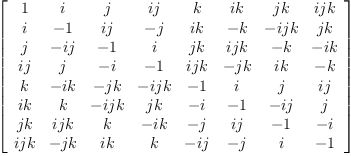(33) Type: Matrix(CaleyDickson(CaleyDickson(CaleyDickson(Fraction(Polynomial(Integer)),i,1),j,1),k,1)) fricas -- -- compare -- Og:ILIST(Octonion R,0):=map(x+-> octon(x.1,x.2,x.3,x.4,x.5,x.6,x.7,x.8), 1$SquareMatrix(8,FRAC INT)::List List FRAC INT)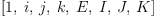(34)
Type: IndexedList?(Octonion(Fraction(Polynomial(Integer))),0)
fricas
matrix [[Og.i * Og.j for j in 0..#Og-1] for i in 0..#Og-1]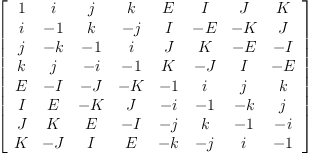(35)
Type: Matrix(Octonion(Fraction(Polynomial(Integer))))
fricas
o1:O := hyper [subscript('o1,[i]) for i in 1..8]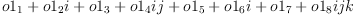(36)
Type: CaleyDickson(CaleyDickson(CaleyDickson(Fraction(Polynomial(Integer)),i,1),j,1),k,1)
fricas
o2:O := hyper [subscript('o2,[i]) for i in 1..8]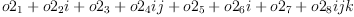(37)
Type: CaleyDickson(CaleyDickson(CaleyDickson(Fraction(Polynomial(Integer)),i,1),j,1),k,1)
fricas
o3:O := hyper [subscript('o3,[i]) for i in 1..8]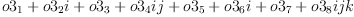(38)
Type: CaleyDickson(CaleyDickson(CaleyDickson(Fraction(Polynomial(Integer)),i,1),j,1),k,1)
fricas
-- normed?
test(norm(o1*o2)=norm(o1)*norm(o2))(39)
Type: Boolean
fricas
-- Commutative?
test( o1 * o2 = o2 * o1 )(40)
Type: Boolean
fricas
-- Associative?
test((o1 * o2) * o3 = o1 * (o2 * o3))(41)
Type: Boolean
fricas
-- Alternative?
test((o1 * o2) * o1 = o1 * (o2 * o1))(42)
Type: Boolean

Split-Octonions

Note: Our table below is not identical the one shown in the reference where a different convention is used to define multiplication.

fricas
sO:=CaleyDickson(Q,'ℓ,-1)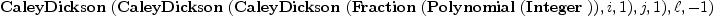(43)
Type: Type
fricas
rank()$sO(44) Type: PositiveInteger? fricas sOe:ILIST(sO,0) := construct entries basis()$sO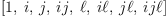(45)
Type: IndexedList?(CaleyDickson(CaleyDickson(CaleyDickson(Fraction(Polynomial(Integer)),i,1),j,1),ℓ,-1),0)
fricas
matrix [[sOe.i * sOe.j for j in 0..#sOe-1] for i in 0..#sOe-1]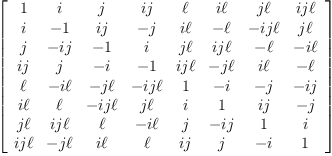(46)
Type: Matrix(CaleyDickson(CaleyDickson(CaleyDickson(Fraction(Polynomial(Integer)),i,1),j,1),ℓ,-1))
fricas
so1:sO := hyper [subscript('so1,[i]) for i in 1..8]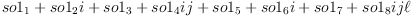(47)
Type: CaleyDickson(CaleyDickson(CaleyDickson(Fraction(Polynomial(Integer)),i,1),j,1),ℓ,-1)
fricas
so2:sO := hyper [subscript('so2,[i]) for i in 1..8]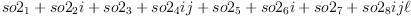(48)
Type: CaleyDickson(CaleyDickson(CaleyDickson(Fraction(Polynomial(Integer)),i,1),j,1),ℓ,-1)
fricas
so3:sO := hyper [subscript('so3,[i]) for i in 1..8]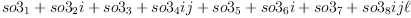(49)
Type: CaleyDickson(CaleyDickson(CaleyDickson(Fraction(Polynomial(Integer)),i,1),j,1),ℓ,-1)
fricas
-- Normed?
test(norm(so1*so2)=norm(so1)*norm(so2))(50)
Type: Boolean
fricas
-- Commutative?
test( so1 * so2 = so2 * so1 )(51)
Type: Boolean
fricas
-- Associative?
test((so1 * so2) * so3 = so1 * (so2 * so3))(52)
Type: Boolean
fricas
-- Alternative?
test((so1 * so2) * so1 = so1 * (so2 * so1))(53)
Type: Boolean
fricas
-- inverse
so1inv := inv so1;
Type: CaleyDickson(CaleyDickson(CaleyDickson(Fraction(Polynomial(Integer)),i,1),j,1),ℓ,-1)
fricas
test(so1 * so1inv = 1)(54)
Type: Boolean

Sedenions

fricas
S:=CaleyDickson(O,'l,1)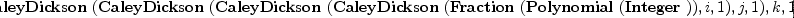(55)
Type: Type
fricas
rank()$S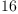(56) Type: PositiveInteger? fricas Se:ILIST(S,0) := construct entries basis()$S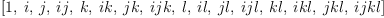(57)
Type: IndexedList?(CaleyDickson(CaleyDickson(CaleyDickson(CaleyDickson(Fraction(Polynomial(Integer)),i,1),j,1),k,1),l,1),0)
fricas
matrix [[Se.i * Se.j for j in 0..#Se-1] for i in 0..#Se-1]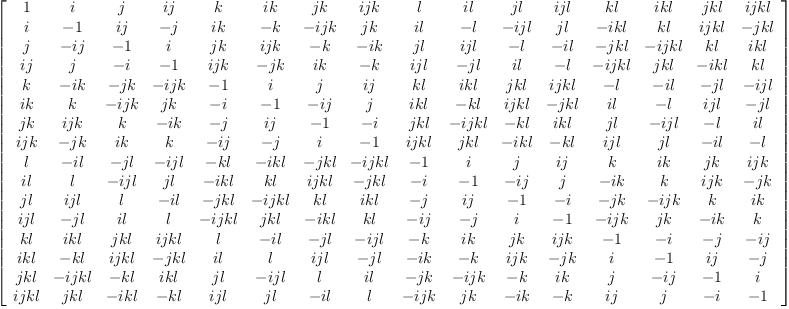(58)
Type: Matrix(CaleyDickson(CaleyDickson(CaleyDickson(CaleyDickson(Fraction(Polynomial(Integer)),i,1),j,1),k,1),l,1))
fricas
s1:S := hyper [subscript('s1,[i]) for i in 1..16]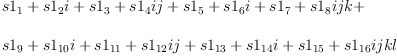(59)
Type: CaleyDickson(CaleyDickson(CaleyDickson(CaleyDickson(Fraction(Polynomial(Integer)),i,1),j,1),k,1),l,1)
fricas
s2:S := hyper [subscript('s2,[i]) for i in 1..16]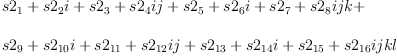(60)
Type: CaleyDickson(CaleyDickson(CaleyDickson(CaleyDickson(Fraction(Polynomial(Integer)),i,1),j,1),k,1),l,1)
fricas
s3:S := hyper [subscript('s3,[i]) for i in 1..16]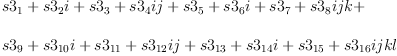(61)
Type: CaleyDickson(CaleyDickson(CaleyDickson(CaleyDickson(Fraction(Polynomial(Integer)),i,1),j,1),k,1),l,1)
fricas
-- normed?
test(norm(s1*s2)=norm(s1)*norm(s2))(62)
Type: Boolean
fricas
-- Commutative
test( s1 * s2 = s2 * s1 )(63)
Type: Boolean
fricas
-- Associative?
test( (s1 * s2) * s3 =  s1 * (s2 * s3) )(64)
Type: Boolean
fricas
-- Alternative?
test((s1 * s2) * s1 = - s1 * (s2 * s1))(65)
Type: Boolean
fricas
-- zero divisor
(Se.3 + Se.10) * (Se.6 - Se.15)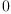(66)
Type: CaleyDickson(CaleyDickson(CaleyDickson(CaleyDickson(Fraction(Polynomial(Integer)),i,1),j,1),k,1),l,1)
fricas
-- inverse
s1inv := inv s1;
Type: CaleyDickson(CaleyDickson(CaleyDickson(CaleyDickson(Fraction(Polynomial(Integer)),i,1),j,1),k,1),l,1)
fricas
test(s1 * s1inv = 1)(67)
Type: Boolean

Power Associative?

Algebra with associative powers

fricas
test( s1^2 * s1 = s1 * s1^2 )(68)
Type: Boolean
fricas
test( (s1^2 * s1) * s1 = s1^2 * s1^2 )(69)
Type: Boolean

Conversions

fricas
test(s1=hyper scalars s1)(70)
Type: Boolean
fricas
scalars(s1)::List Symbol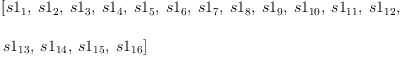(71)
Type: List(Symbol)

 Subject:   Be Bold !! ( 14 subscribers )